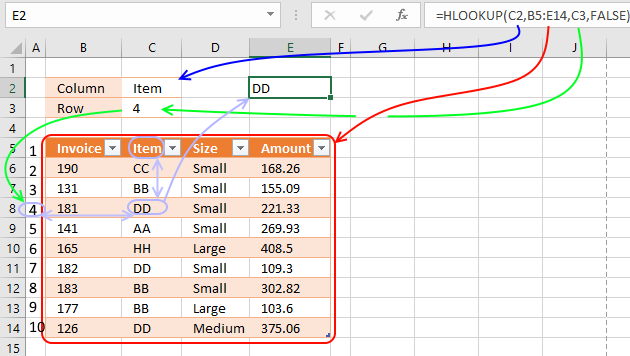Author: Oscar Cronquist Article last updated on April 16, 2018

####The HLOOKUP function lets you search the top row in a data range for a value and return another value on the same column in a row you specify.

HLOOKUP stands for horizontal lookup and your data (table_array) must be organized into records, a record on each row.

To search records excel must look horizontally in the top row of the data array. VLOOKUP stands for vertical lookup but that is another post.

Formula in cell E2:

=HLOOKUP(C2,B5:E14,C3,FALSE)

The HLOOKUP function in cell E2 searches the top row of cell range B5:E14 for value "Item" and it is found in the second column.

The third argument tells the function which row to fetch the value from, in this case it is four.

The intersection of the second column and the fourth row is value DD and that is the returning value in cell E2.

### Excel Function Syntax

HLOOKUP(lookup_value, table_array, col_index_num, [range_lookup])

### Arguments

 Lookup_value Required. The first argument is the lookup value. This is the value you want to find in the top row of the table array. Table_array Required. This is a cell range or an array that you want to search. Row_index_num Required. Here you specify which row you want fetch the value from. Range_lookup Optional. You may select TRUE or FALSE here, TRUE tells the function to do an approximate match and this is the default value.

Make sure the top row in the table array is sorted ascending. FALSE means exact match and this mode is probably what you will use the most. Take note that if you use the wrong argument here you may get incorrect results.

#### Approximate match

The HLOOKUP function allows you to search with an approximate match if you enter TRUE in the fourth argument. In the example below there are four different ranges, 0 and less than 100, 100 and less than 250, 250 and less than 500 and 500 and more.

The value 189 in cell C2 can't be found in the top row of C5:F6 so the next largest value that is less than lookup_value (189) is matched, in this case 100. The corresponding value is 12.1 and is returned in cell C3.

#### Horizontal and vertical lookup

The following formula lets you search both horizontally and vertically. The formula in cell E2 looks for Item in the top horizontal row and 141 in column "Invoice", the intersecting cell is C9 so the formula returns AA.

Formula in cell E2:

=HLOOKUP(C2,B5:E14,MATCH(C3,B5:B14,0),FALSE)

#### Horizontal and vertical lookup - INDEX + MATCH

You can also use INDEX and MATCH functions to get a value in a cross reference table. The formula below works just like the formula above.

Formula in cell E2:

=INDEX(B6:E14,C3,MATCH(C2,B5:E5,0))

This formula is actually easier to understand and remember than the HLOOKUP function, this is the method I prefer.

#### HLOOKUP - multiple tables

The formula below lets you also choose a table to search. The INDIRECT function lets you convert a cell value to a cell reference, however the INDIRECT function is volatile, so use it with care.

Volatile means it is recalculated every time the worksheet is recalculated. If you have many INDIRECT formulas, they may slow down your workbook.

Formula in cell J4:

=HLOOKUP(H4,INDIRECT(G4),I4,FALSE)

#### HLOOKUP - VBA Example

The following macro lets you search a table using the parameters in cell C2 and C3. A messagebox appears and tells you the returning value.

VBA Code

```Sub HLP()
MsgBox Application.WorksheetFunction.HLookup(Range("C2"), Range("B5:E14"), Range("C3"), False)
End Sub
```